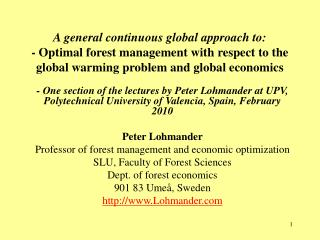DownloadDownload PresentationSecond order minimum conditions:

# Second order minimum conditions:

Download Presentation## Second order minimum conditions:

- - - - - - - - - - - - - - - - - - - - - - - - - - - E N D - - - - - - - - - - - - - - - - - - - - - - - - - - -
##### Presentation Transcript

1. A general continuous global approach to:- Optimal forest management with respect to theglobal warming problem and global economics - One section of the lectures by Peter Lohmander at UPV, Polytechnical University of Valencia, Spain, February 2010 Peter Lohmander Professor of forest management and economic optimization SLU, Faculty of Forest Sciences Dept. of forest economics 901 83 Umeå, Sweden http://www.Lohmander.com

2. Second order minimum conditions:

3. Observations of the first and second order conditions:

4. So, if and and or or then

5. Then, the solution to represents a (locally) unique minimum.

6. A numerically specified example:

7. Comparative statics analysis:

8. Explicit solution of the example for alternative values of K

9. Dynamic approach analysis

10. k is selected in way such that the two equations become identical. This way, the equations only determine the ratio B/A, not the values of A and B. This is necessary since we must have some freedom to determine A and B such that they fit the initial conditions. • With two roots (that usually are different), we (usually) get two different ratios B/A. This makes it possible to fit the parameters to the (two dimensional) initial conditions (x(0),y(0)).

11. Another way to get to the same equation, is to make sure that the two equations give the same value to the ratio B/A.

12. Lets us solve the equation!

13. No cyclical solutions! • Observe that the expression within the square root sign is positive. • As a consequence, only real roots, k, exist. • For this reason, cyclical solutions to the differential equation system can be ruled out.